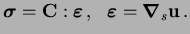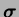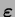Next: Implementation of the Natural Up: Natural Neighbor Interpolation Previous: Natural Neighbor Interpolation

## Governing Equations and Weak Form

In order to study a model PDE, let us consider small displacement elastostatics, which is governed by the equation of equilibrium:in(7)

where(8)

In the above equations,is the domain of the body,is the Cauchy stress tensor,is the small strain tensor,is the body force per unit volume,is the material moduli tensor,is the displacement,is the gradient operator, andis the symmetric gradient operator.

The essential and natural boundary conditions areonon(9)

whereis the boundary of, andandare prescribed displacements and tractions, respectively.

The weak form (principle of virtual work) is(10)

On substituting the trial and test functions in the above equation and using the arbitrariness of nodal variations, the following discrete system of equations is obtained:(11)

where(12)

In the above equation,is the shape function vector andis the matrix of shape function derivatives.Next: Implementation of the Natural Up: Natural Neighbor Interpolation Previous: Natural Neighbor Interpolation
N. Sukumar